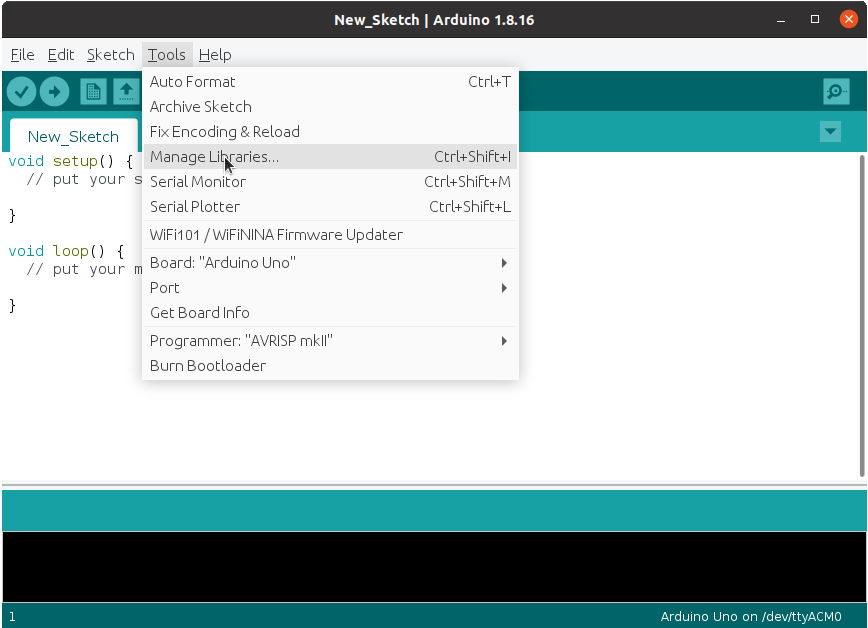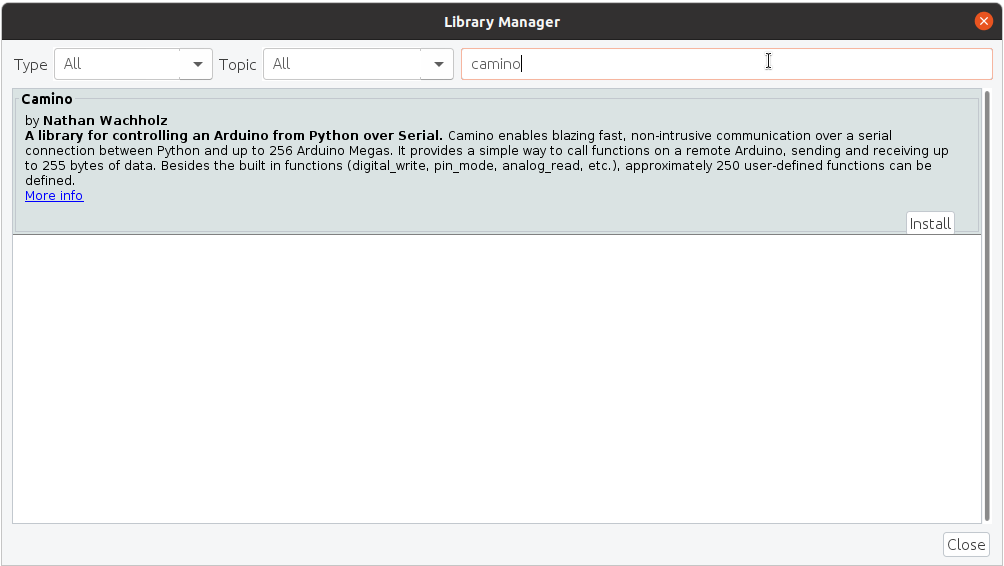# Controlling an Arduino from Python

There are a lot of tutorials online explaining how to control an Arduino from a computer using Python. Unfortunately, many of them are outdated (for Python 2), don't work on Windows/Linux, or are hard to extend to your use case. In this post, I'll show you how to control an Arduino from Python using `camino`, which offers fast performance over a simple API.

Perhaps the most important fact about `camino` is that we don't need to do anything special in our `loop` function: `camino` runs entirely in the background!

## Getting Started

To get started, we'll install `camino` on our computer and upload the `CaminoExample` sketch to our Arduino. This will allow us to perform basic operations like `pinMode`, `digitalRead` and `digitalWrite`.

First, open up the Arduino IDE and connect your Arduino. Then, install the `Camino` library from the Arduino Library Manager:Then, open the `CaminoExample` sketch, and upload it to your Arduino:Take note of the port that your Arduino is connected to. You'll need this later.

To install `camino` on your computer, run:

``````pip install camino
``````

## Basic Usage

Now that we have everything installed, we can start using `camino` to control our Arduino. Make sure that your Arduino is plugged in, with the `CaminoExample` sketch uploaded. From Python, we will connect to the Arduino, and then make the builtin LED blink.

First, we create an `Arduino` object that is connected to the right port.

``````from camino import SerialConnection, Arduino
connection = SerialConnection(port="/dev/ttyACM0")  # Replace with your port from earlier
arduino = Arduino(connection)
``````

Now, we can run some basic commands. For example, we can set the builtin LED to be an output:

``````arduino.pin_mode(13, 1)  # 1 is OUTPUT, 0 is INPUT
``````

Then, we can make it blink using `digital_write`, and Python's `time.sleep`.

``````while True:
arduino.digital_write(13, 1)
time.sleep(0.5)
arduino.digital_write(13, 0)
time.sleep(0.5)
``````

When running the `CaminoExample`, you have access to the following built-in functions:

• `pin_mode(pin, mode)`
• `digital_read(pin, out=int)`
• `digital_write(pin, value)`
• `analog_read(pin, out=int)`
• `analog_write(pin, value)`

If you want to do something more complicated, you'll need to add the function to the Arduino sketch.

## Adding new Arduino functions to run from Python

As an example, let's add a function to the Arduino sketch that will do some math. Specifically, we'll add a function that takes in a number, and returns the square of that number.

First, we'll add the function to the Arduino sketch. Open up the `CaminoExample` sketch, and add the following function:

``````void square(byte length, byte* data) {
int x = data; // get the first argument
int y = x * x;
returns(y);
}
``````

Then, at the bottom of the file, we'll add the function to the list of `CALLABLES`. This is how `camino` knows how to call the function from Python. Modify the `CALLABLES` section to include `square`:

``````BEGIN_CALLABLES {
{"say_hi", sayHi},
{"square", square}, // the first argument is the name to use in Python
} END_CALLABLES;
``````

Then, upload the sketch to the Arduino. Now, we can use this function from Python!

``````# Recreate the Arduino so it refreshes the commands
arduino = Arduino(connection)
# then run our new function
arduino.square(5, out=int)  # returns 25
``````

This is just a simple example, but it illustrates that you can add your own functions to run from Python.

And remember, you don't need to do anything special in your `loop` function, as `camino` runs entirely in the background! You can use `camino` to talk to your Arduino (like check if a button is pressed), while still using the Arduino's `loop` function to do other things.

To add `camino` to an existing sketch, you'll need to `#include <Camino.h>` at the top of your sketch, add a call to `camino.begin(9600)` in `setup`, and then add the `CALLABLES` section to the bottom of your sketch. It can be empty if you only want to use the built-in functions.

## Conclusion

In this post, we saw how to use `camino` to control an Arduino from Python. We saw how to use the built-in functions, and how to add new functions to the Arduino sketch. `camino` can do a lot more, so check out the documentation. And if you have any questions or issues, feel free to open an issue on GitHub.